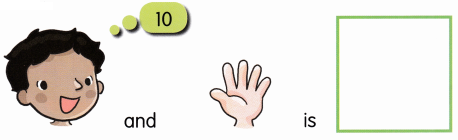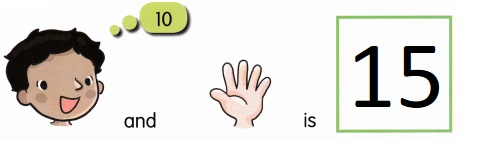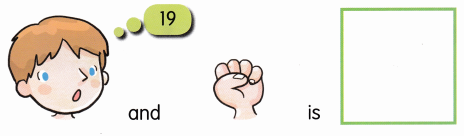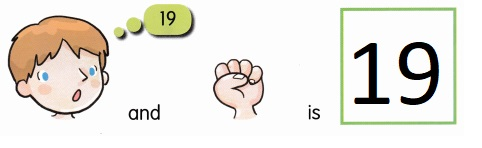# Math in Focus Kindergarten Chapter 9 Answer Key Comparing Sets

Go through the Math in Focus Grade K Workbook Answer Key Chapter 9 Comparing Sets to finish your assignments.

## Math in Focus Kindergarten Chapter 9 Answer Key Comparing Sets

Lesson 1 Comparing Sets of Up to 10

Count and Write.

Question 1.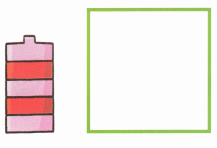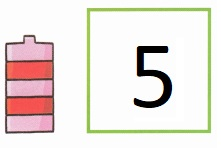Explanation:
Counted 5, wrote the number in the given space.

Question 2.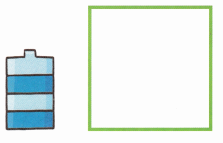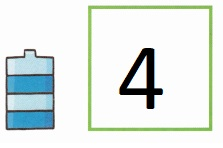Explanation:
Counted 4, wrote the number in the given space.

Question 3.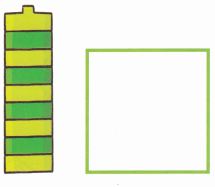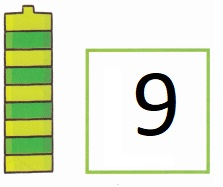Explanation:
Counted 9, wrote the number in the given space.

Question 4.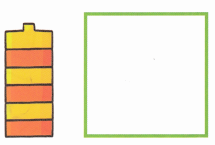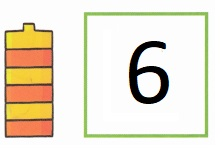Explanation:
Counted 6, wrote the number in the given space.

Which has more? Color. Which has fewer? Circle.

Question 1.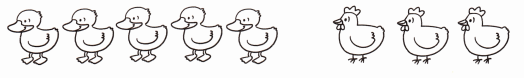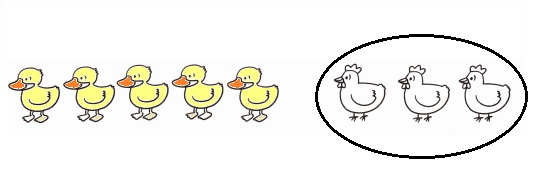Explanation:
Colored 5 ducks which are more and circled fewer 3 cocks .

Question 2.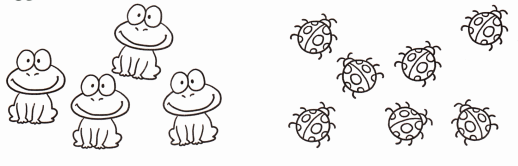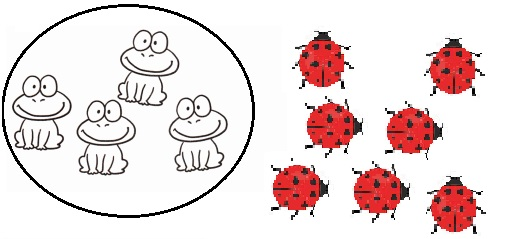Explanation:
Colored 7 bees which are more and circled fewer 4 frogs .

Question 3.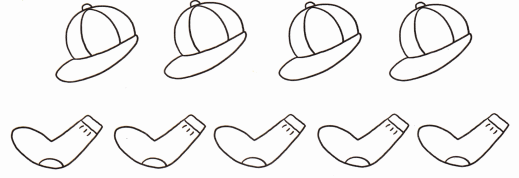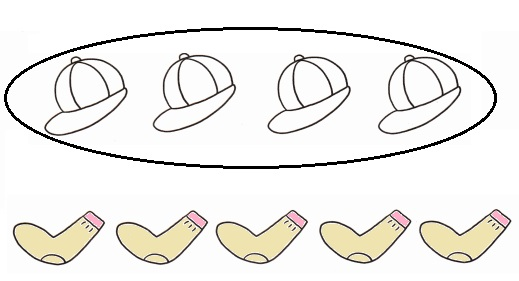Explanation:
Colored 5 socks which are more and circled fewer 4 caps.

What cannot be counted? Circle.

Question 1.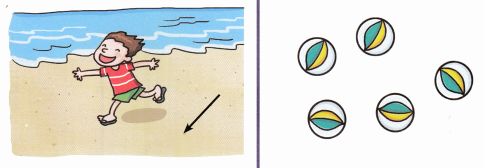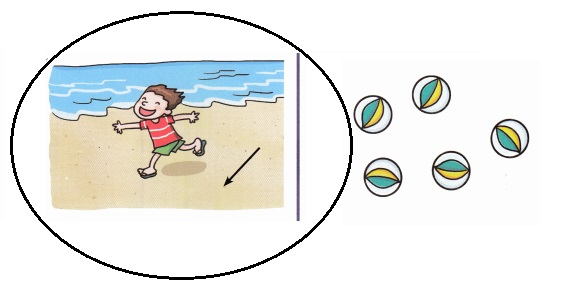Explanation:
Seashore cannot counted.

Question 2.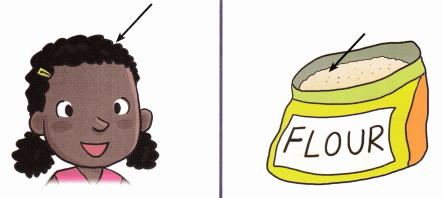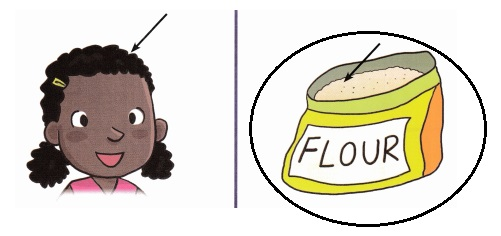Explanation:
Flour cannot counted.

Question 3.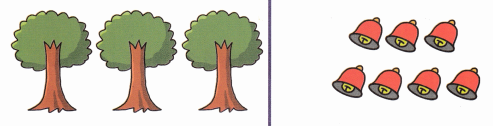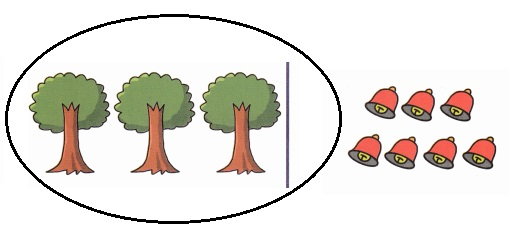Explanation:
Tree leaves cannot counted.

Lesson 2 Comparing Sets of 11 to 20

Count and write. Circle the set with more.

Question 1.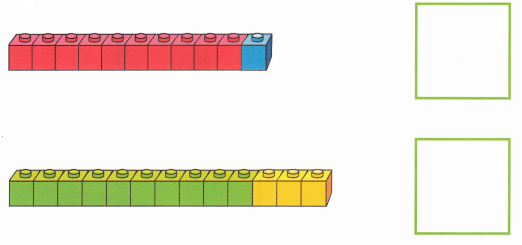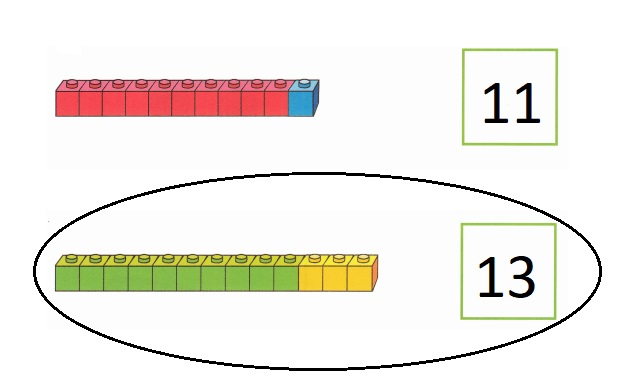Explanation:
Counted 11, 13 and wrote. Circled the set 13 with more.

Question 2.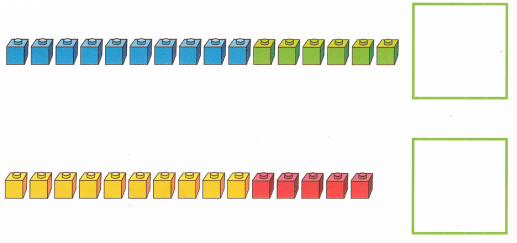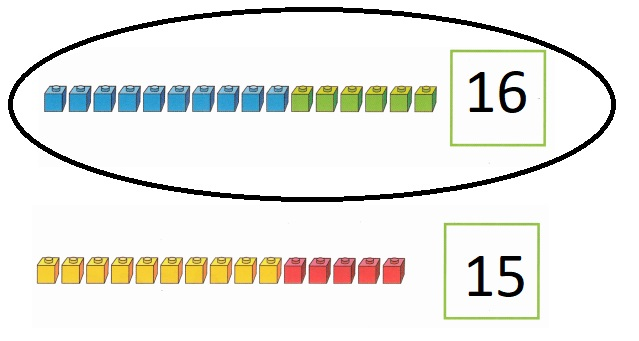Explanation:
Counted 16, 15 and wrote. Circled the set 16 with more.

Match one-to-one. Then, color the set with fewer.

Question 1.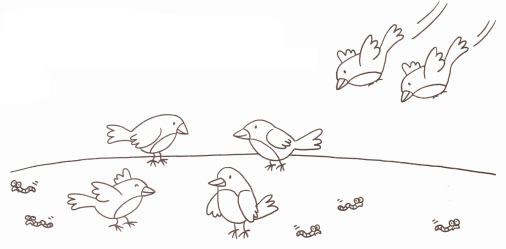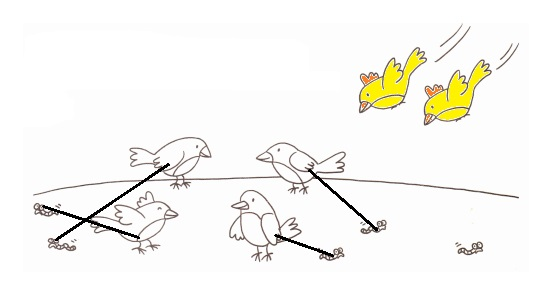Explanation:
Matched one-to-one. Then, colored the set with fewer.

Question 2.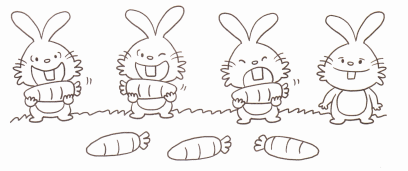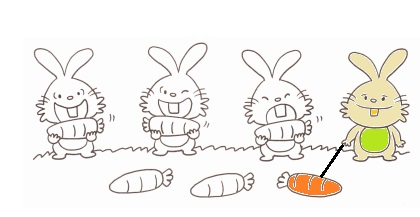Explanation:
Matched one-to-one. Then, colored the set with fewer.

Question 3.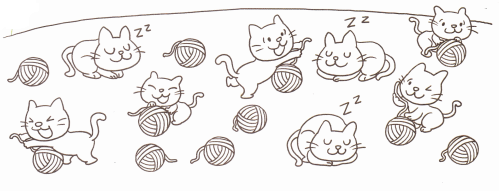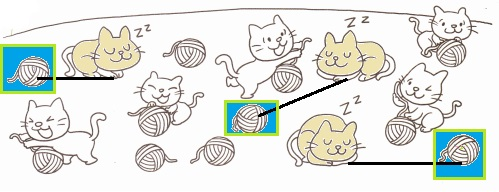Explanation:
Matched one-to-one. Then, colored the set with fewer.

Lesson 3 Comparing Sets to Find the Difference

Color the extra cubes red. Count and write how many more.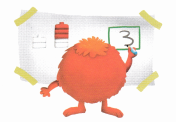Question 1.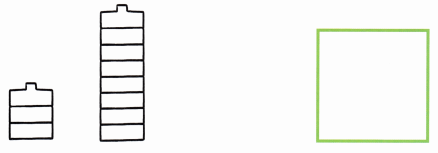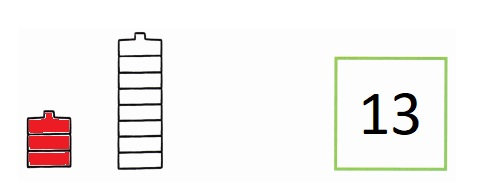Explanation:
Colored the extra 3 cubes with red. Counted 13 and wrote.

Question 2.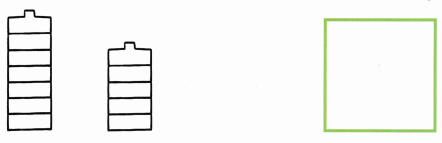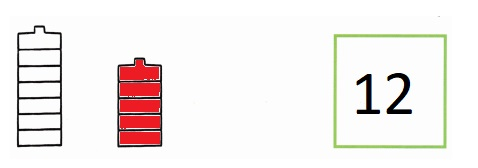Explanation:
Colored the extra 5 cubes with red. Counted 12 and wrote.

Question 3.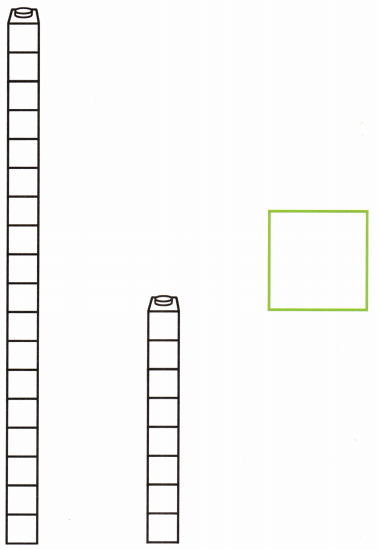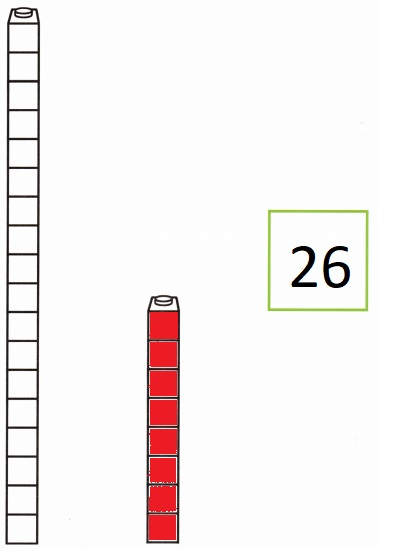Explanation:
Colored the extra 8 cubes with red. Counted 26 and wrote.

Draw, count, and write.

Question 1.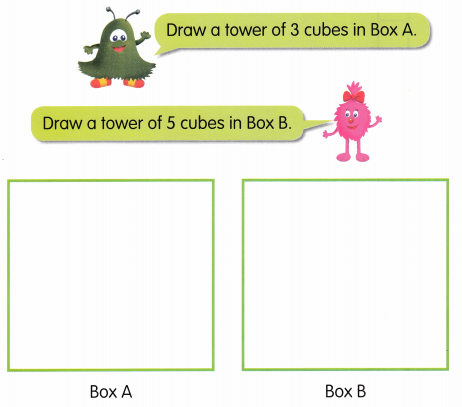The tower in Box A has ____2_____ fewer cubes than the tower in Box B.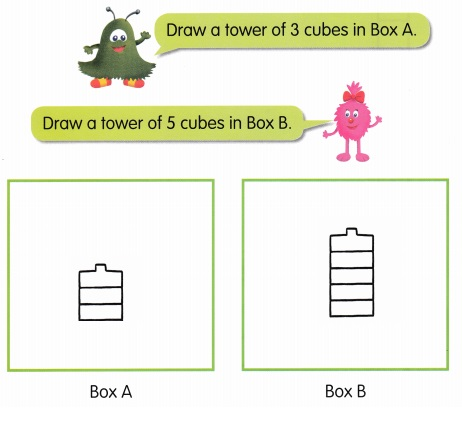Explanation:
Drawn a tower of 3 cubes in Box A and drawn a tower of 5 cubes in Box B,
The tower in Box A has 2 fewer cubes than the tower in Box B.

Question 2.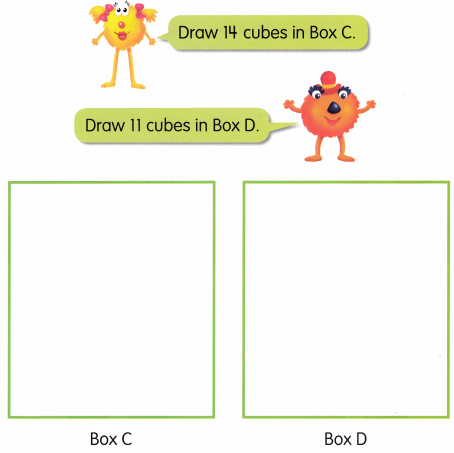Box C has ____3_______ more cubes than Box D.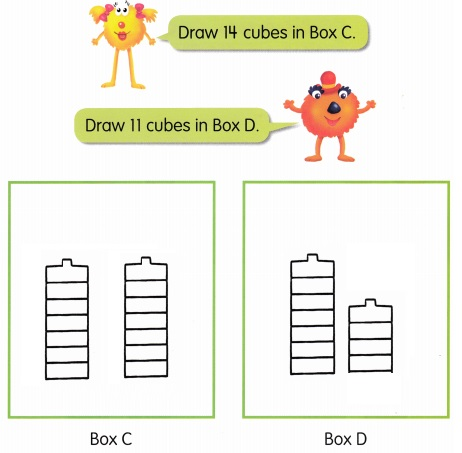Explanation:
Drawn a tower of 14 cubes in Box  C and drawn a tower of 11 cubes in Box D,
The tower in Box C has 3 fewer cubes than the tower in Box D.

Lesson 4 Combining Sets

Count and circle.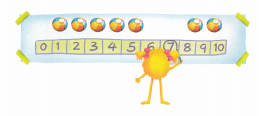Question 1.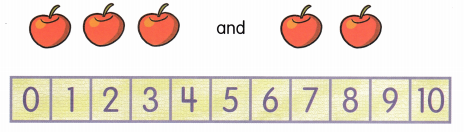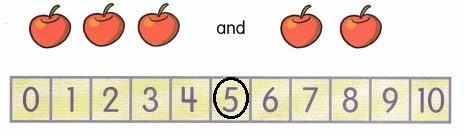Explanation:
Counted 3 and 2 we circled 5.

Question 2.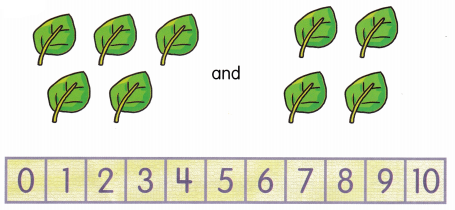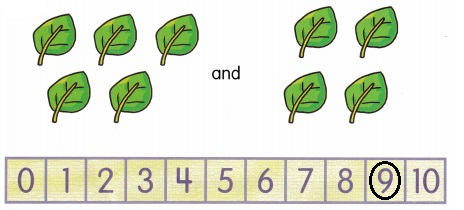Explanation:
Counted 5 and 4 we circled 9.

Question 3.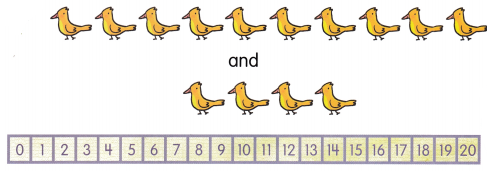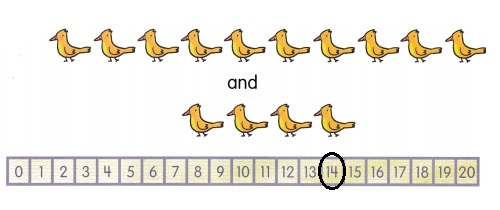Explanation:
Counted 10 and 4 we circled 14.

Question 4.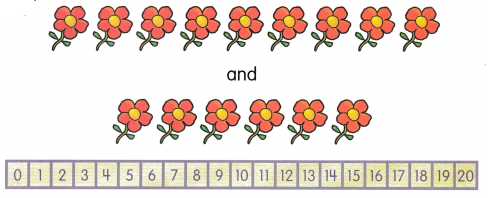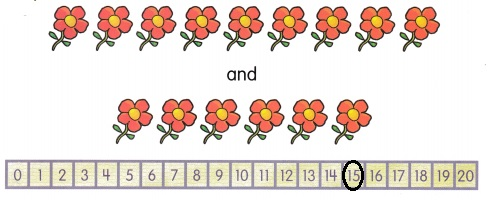Explanation:
Counted 9 and 6 we circled 15.

Question 5.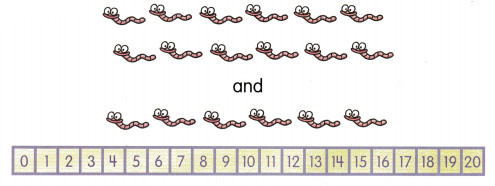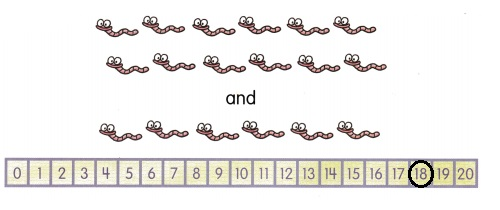Explanation:
Counted 12 and 6 we circled 18.

Count, circle, and write.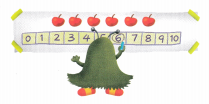Question 1.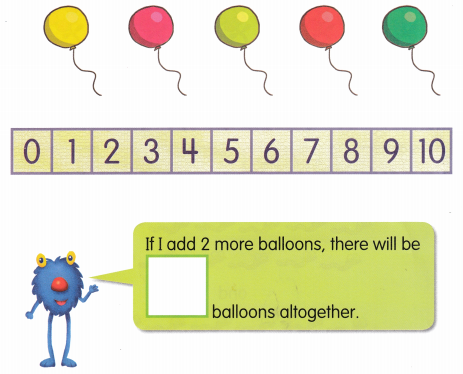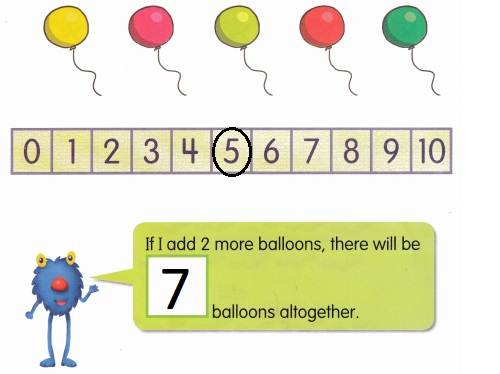Explanation:
Counted 5 balloons and circled 5 balloons,
If I add 2 more balloons to 5, there will be 7 balloons altogether.

Question 2.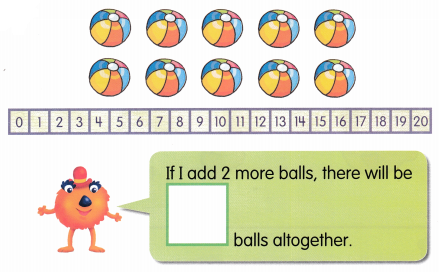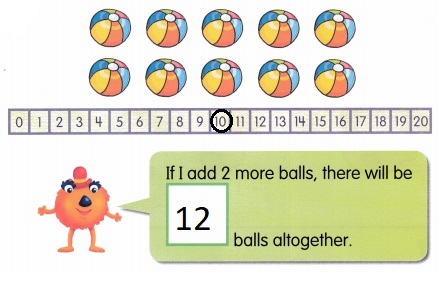Explanation:
Counted 10 balls and circled 10 balls,
If I add 2 more balls to 10 balls, there will be 12 balls altogether.

Question 3.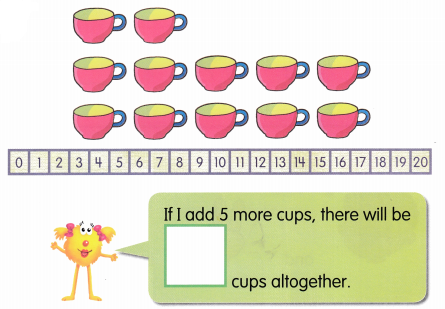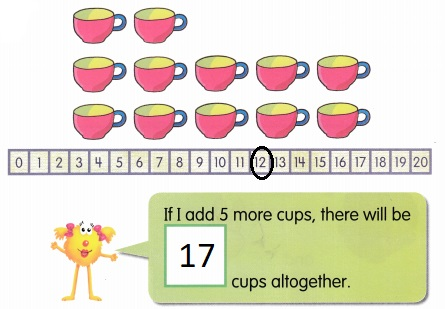Explanation:
Counted 12 cups and circled 12 cups,
If I add 5 more cups to 12 cups, there will be 17 cups altogether.

Count and write.

Question 1.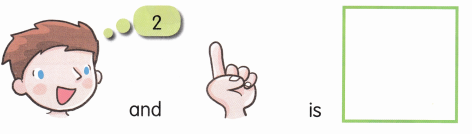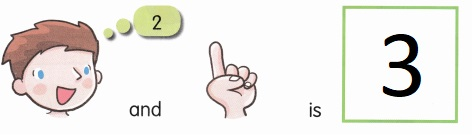Explanation:
2 and 1 more is 3.

Question 2.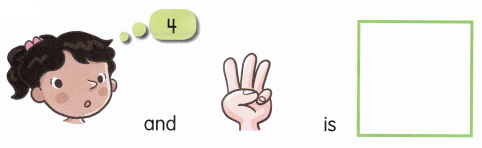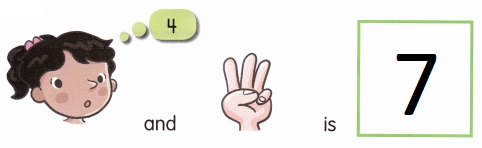Explanation:
4 and 3 more is 7.

Question 3.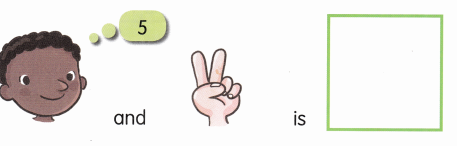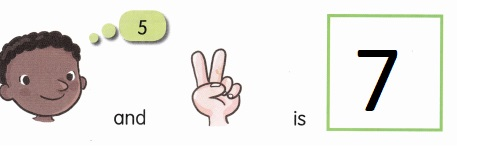Explanation:
5 and 2 more is 7.

Question 4.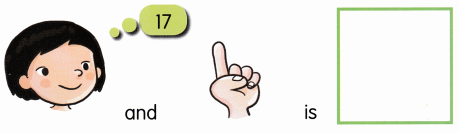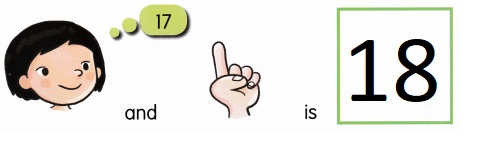Explanation:
17 and 1 more is 18.

Question 5.